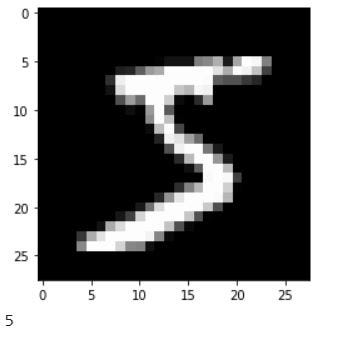# Digit Recognition on MNIST dataset using Convolution Neural Network (CNN)

Original article was published on Deep Learning on Medium# Digit Recognition on MNIST dataset using Convolution Neural Network (CNN)

The main objective of this post is to make people understand the mostly viewed information on how to implement the basic neural network model used for classification or recognition using MNIST dataset.

MNIST Dataset

The MNIST dataset of digits is available on the link, it has a training set of 60,000 examples, and a test set of 10,000 examples.
It is a good database for people who want to try various techniques and pattern recognition methods while spending minimal efforts on preprocessing and formatting.

1. The MNIST Database — http://yann.lecun.com/exdb/mnist/
2. Tensorfow.org — https://www.tensorflow.org/datasets/catalog/mnist

Now lets start coding:

# Import the libraries:

`#Used for Imagesimport cv2#contains a multi-dimentional array and matrix data structures, mathematical operationsimport numpy as np#Loads the MNIST datasetfrom keras.datasets import mnist#Regular densely-connected NN layer; Flattens the input; Convolution 2-Dimentionalfrom keras.layers import Dense, Flattenfrom keras.layers.convolutional import Conv2D#Sequential class which is a linear stack of Layersfrom keras.models import Sequential#Converts a class vector (integers) to binary class matrix.from keras.utils import to_categorical#creates a figure, creates a plotting area in a figure, plots some lines in a plotting area, decorates the plot with labelsimport matplotlib.pyplot as plt`

# Import MNIST Dataset:

`#Loads MNIST dataset and divides the data in train and test.(X_train, y_train), (X_test, y_test) = mnist.load_data()`

# Random display of one image with the label from the dataset:

`#Displaying any one image from the dataset in gray scale.plt.imshow(X_train, cmap=”gray”)plt.show()print (y_train)`

When we run the above code we will get the gray scale image as well as the label as shown below:

# Checking the shape of the MNIST Dataset:

The shape of the MNIST database contains 28,28 as the image size and 60000 represents the training data.

Output of the above code:
Shape of X_train: (60000, 28, 28)
Shape of y_train: (60000,)
Shape of X_test: (10000, 28, 28)
Shape of y_test: (10000,)

# Reshaping and checking the data of our model:

`#Reshaping the data for the model.#In the output 60000 represents training data; 28,28 represents the image size and 1 represents that it is a gray scale image.X_train = X_train.reshape(60000, 28, 28, 1)X_test = X_test.reshape(10000, 28, 28, 1)`

In the output 60000 represents training data; 28,28 represents the image size and 1 represents that it is a gray scale image.

`#Checking the shape of the training and test data according to the model.print ("Shape of X_train: {}".format(X_train.shape))print ("Shape of y_train: {}".format(y_train.shape))print ("Shape of X_test: {}".format(X_test.shape))print ("Shape of y_test: {}".format(y_test.shape))`

Output of the above code:
Shape of X_train: (60000, 28, 28, 1)
Shape of y_train: (60000,)
Shape of X_test: (10000, 28, 28, 1)
Shape of y_test: (10000,)

# Converting to binary class

`#Converts a class vector (integers) to binary class matrix.y_train = to_categorical(y_train)y_test = to_categorical(y_test)`

# Build the Sequential Model

`# Declare the Sequential modelmodel = Sequential([ Conv2D(32, kernel_size=3, activation=’relu’, input_shape=(28, 28, 1)), Conv2D(64, kernel_size=3, activation=’relu’), Flatten(), Dense(10, activation=’softmax’)])#Display the above modelmodel.summary()`

Output of the above model:

# Compiling the model:

`#Training the model for the test validation data based on trained data# epochs is the number of times all of the training vectors are used once to update the weights which is set to 3model.fit(X_train, y_train, validation_data=(X_test, y_test), epochs=3)`

Output: